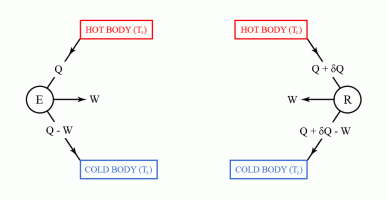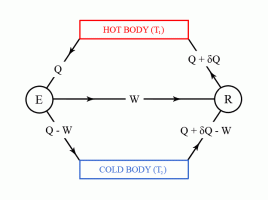•http://facebook.com/
•https://www.google.com/accounts/o8/id
•https://me.yahoo.com

# Second Law of Thermodynamics

An introduction to the second law of thermodynamics, and discussing Carnot's theorem.

## Overview

Definitions

Heat engine is any machine that can convert heat into work and vice versa.

Available energy is the proportion of heat supplied to a machine which can be converted into mechanical work. This proportion is found to be dependent on the temperature of the heat source, and also on the temperature at which heat is rejected.

<br/>

Key facts

The second law of thermodynamics states that heat can not be transferred from a body at a lower temperature to a body at a higher temperature without the application of energy from an external source.

Carnot's theorem states that no machine can be more efficient than a machine working under a thermodynamically reversible cycle, provided that they are both working under the same limits of temperature.

Carnot's theorem can be proved by reductio ad absurdum using the second law of thermodynamics.

The second law of thermodynamics states that heat can not be transferred from a body at a lower temperature to a body at a higher temperature without the application of energy from an external source.

## Carnot's Theorem

The second law of thermodynamics can be used to prove Carnot's theorem. However, before discussing Carnot's theorem, we first have to introduce the thermal efficiency of a heat engine.

The thermal efficiency of a heat engine, also denoted by , is a means of comparing the ability of an engine to convert heat energy into work. Therefore, the thermal efficiency can be defined as:

where is the mechanical work output, and the heat energy supplied.

As the first law of thermodynamics states that the energy output can not exceed the energy input (for a more detailed discussion see First Law of Thermodynamics ), we have:

or, expressed as percentages:

Examples of thermal efficiencies are 4% for steam locomotives, 18%-30% for internal combustion engines, and 30% for modern power stations. From these examples it can be seen that the conversion of heat into work involves considerable losses in the available energy, and indeed, the efficiencies of most heat engines are generally much less than 100%.

Carnot's theorem states that no machine can be more efficient than a machine working under a thermodynamically reversible cycle, provided that they are both working under the same limits of temperature.

In order to prove Carnot's theorem, consider two engines and - working under an irreversible cycle, and working under a reversible cycle. Also, assume by reductio ad absurdum that is more efficient than .

Consider that initially takes units of heat at temperature , and converts into work, while units are rejected to a heat sink at temperature (see Figure 1, left side). The thermal efficiency of engine can thus be written as:

At the same time, engine takes units of heat at temperature , and converts into work, while units are rejected to a heat sink at temperature (see Figure 1, right side). The thermal efficiency of engine is thus given by:

and as stated in the hypothesis, is less than that of engine .Figure 1 We then consider the reverse process in the reversible engine . Thus, takes units of work to drive it backwards, and rejects units at temperature (see Figure 2).Figure 2 Now imagine that the work output of engine is used to provide the power to drive backwards (see Figure 3).Figure 3 As can be seen from Figure 3, in these conditions units of heat will be transferred from the cold sink to the hot sink. Considering that this is an isolated system within which no external work is being achieved, this would then contradict the second law of thermodynamics. Thus, the original assumption can not be true.

Therefore, indeed, there can not exist a machine more efficient than one working under a thermodynamically reversible cycle, provided that they are both working under the same limits of temperature (Carnot's theorem).

Last Modified: 16 Oct 11 @ 05:37     Page Rendered: 2022-03-14 16:01:22